# Concrete Calculator Formula Forfiguring concrete yardage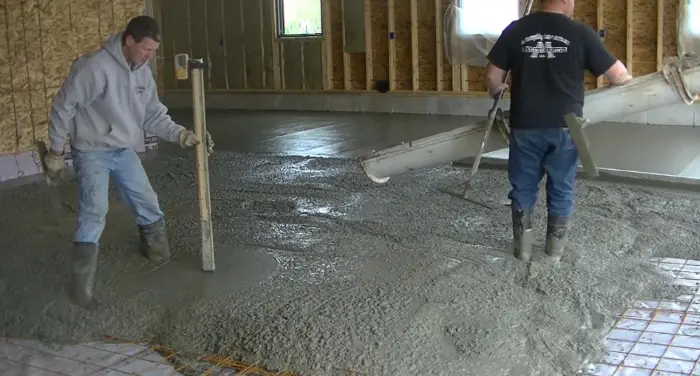The concrete calculator formula to figure cubic yards for a square or rectangle shaped concrete slab is (Length x Width x Thickness) / 27. Convert all numbers to feet before doing the calculation.

Below you'll find a written formula to calculate concrete yardage for concrete projects like:

• Concrete slabs
• Round slabs
• Concrete walls
• Footings
• Columns (square and round)
• Concrete steps
• Curbs and Gutters

## Concrete calculator formula for floors and slabs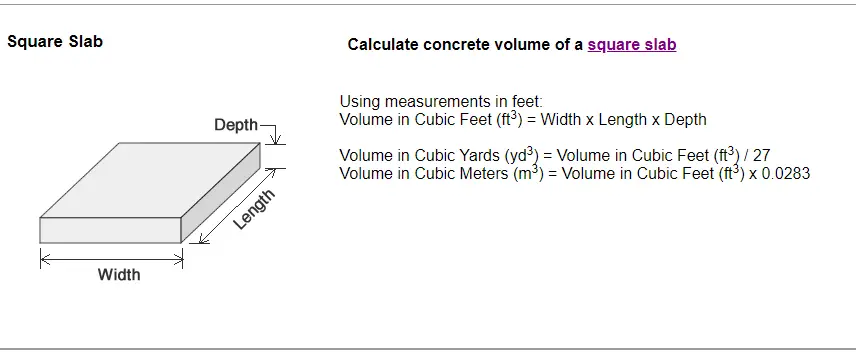This is how you use a formula to calculate concrete for a square concrete slab or a rectangular shaped floor or slab.

Concrete volume in cubic feet (ft3) = Width x Length x Depth

(depth is converted to feet by dividing inches / 12)

Total Cubic Yards (yd3) = Volume in cubic feet (ft3) / 27

(27) is the number of cubic feet in 1 cubic yard of concrete.

How to convert volume to cubic meters (M3)

Volume in cubic meters (M3) = Volume in cubic feet (ft3) x 0.0283

EXAMPLE: 20' X 20' X 4" SLAB

20' x 20' = 400 x .33' = 132 cubic feet

132 / 27 = 4.89 cubic yards of concrete

132 x 0.0283 = 3.74 cubic meters of concrete

[Twenty seven (27) is the number of cubic feet in 1 yard of concrete 3x3x3]

LEARN HOW TO POUR YOUR OWN CONCRETE SLAB HERE

## concrete calculator formula for a round slab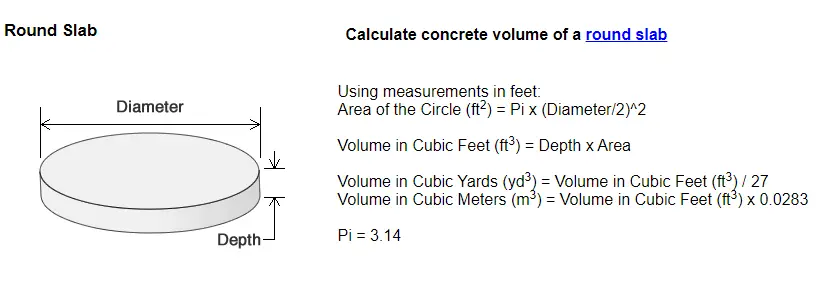The formula to calculate concrete yardage for a round slab is very different than the formula for a square slab. Square footage is calculated using Pi and the diameter of the circle to help determine cubic yardage.

Example: Diameter = 20' (feet)  and depth = 4" (inches)

Area in square feet equals  3.14 x (20÷2)²  which equals 3.14 x 100 = 314 sq. ft.

Volume in cubic feet = .33 x 314 = 103.62

Volume in cubic yards = 103.63 ÷ 27 = 3.84 cubic yards of concrete

Volume in cubic meters = 103.62 x .0283 = 2.93 cubic meters of concrete

## calculator formula for a concrete wall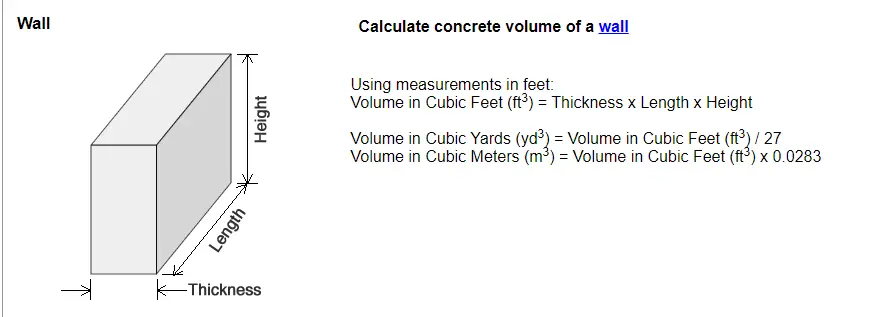Calculating concrete yardage for a wall uses the same formula as a slab.

Example: Height = 8', Length = 40', Thickness = 8"

Convert thickness to feet: 8 ÷ 12 = 0.667 feet

Volume in cubic feet = .667 x 8 x 20 = 213.44 ft³

Volume in cubic yards = 213.44 ft³ ÷ 27 = 7.9 yd³

Volume in cubic meters = 213.44 ft³ x .0283 = 6.04 m³

## Formula to calculate concrete for a footer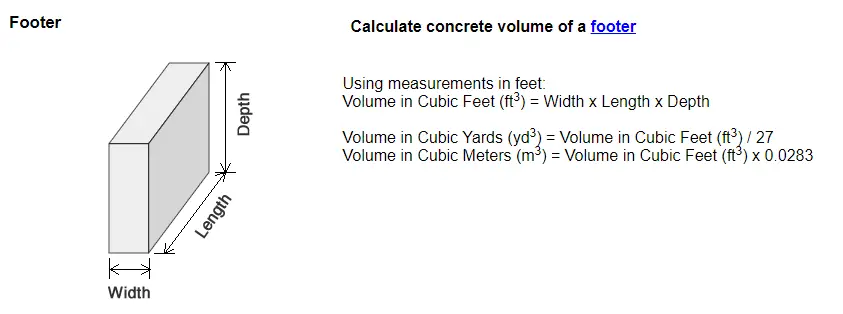Calculating concrete yardage for a footing is done much like a slab, you just usually have smaller units of measurement for width.

Example: Width 18", Depth 8", Length 50' (convert inches to feet)

Volume in Cubic feet = 1.5 x .66 x 50 = 49.5 cubic feet

Volume in Cubic yards = 49.5 ÷ 27 =  1.83 cubic yards

Volume in cubic meters = 49.5 x .0283 = 1.4 cubic meters

## Concrete calculator formula for a square column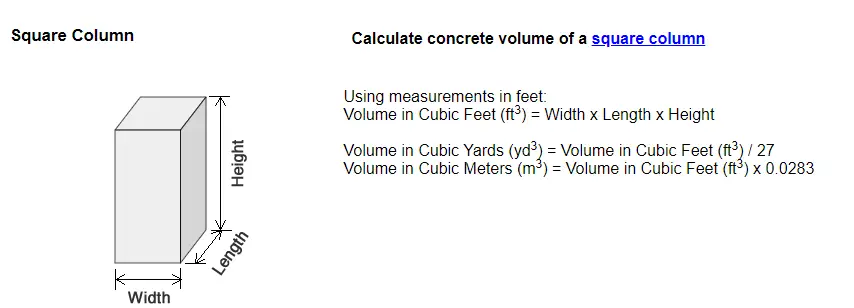Calculating concrete for a square column first starts with calculating the cubic feet of the column.

Example: Width = 2', Length = 3' Height = 18'

To calculate cubic feet multiply 2 x 3 x 18 that equals 108 cubic feet

To calculate cubic yards divide 108 by 27, that equals 4 yd³

To calculate cubic meters of concrete, multiply cubic feet 108 by .0283 = 3.05 M³

## Formula to calculate concrete for a round column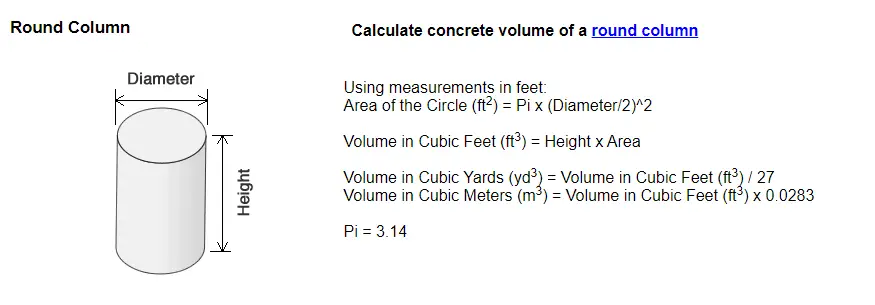The formula to calculate concrete yardage for a round column is just like the round slab formula above. The difference is a column's height is usually much greater than a slab.

Example: Diameter 3', Height 30'

Area of the circle in square feet is 3.14 x (3÷2)² = 7.065 ft²

Volume in ft³ = 30 (H) x 7.065 (A) = 211.95 ft³

Volume in yd³ = 211.95 ft³ ÷ 27 = 7.85 yd³

Volume in m³ = 211.95 ft³ x .0283 = 5.99 m³ of concrete

## How to calculate concrete for steps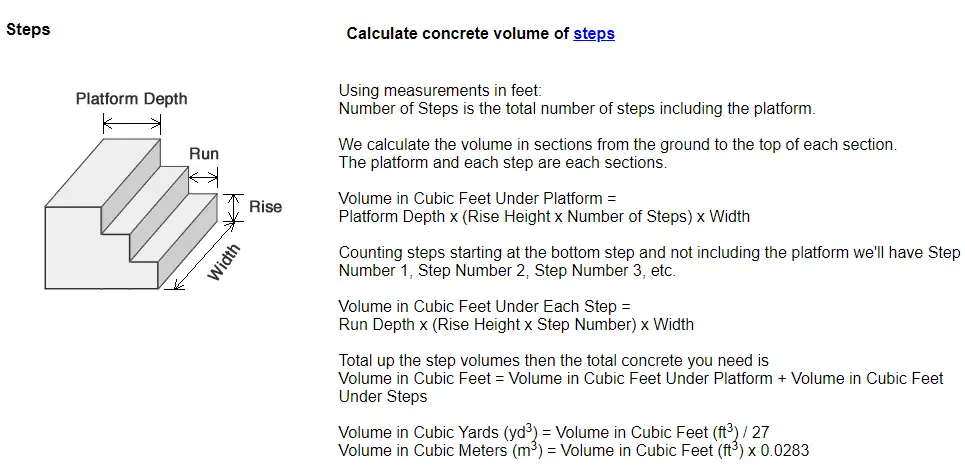This is how you calculate concrete yardage for a set of concrete steps.

Example: Platform Depth 3', Width 6', Run 1', Rise 8".

1st calculate cubic feet for each step. In the example above the first step is:

Run depth = 1' x (Rise height) .66' x 1 (step #1) x 6 (step width) = 3.96 ft³ for step #1

2nd Step is:

Run depth = 1' x (Rise height) .66' x 2 (step #2) x 6 (step width) = 7.92 ft³ for step #2

Platform is:

Platform depth = 3' x (Rise height) .66 x 3 (total steps) x 6 (step width) = 35.64 ft³ for platform

Total cubic feet volume = 35.64 + 7.92 + 3.96 = 47.52 ft³

Cubic yard volume = 47.52 ft³ ÷ 27 = 1.76 yd³ of concrete

Cubic meter volume = 47.52 ft³ x .0283 = 1.34 m³ of concrete

## formula to calculate concrete for curbs and gutters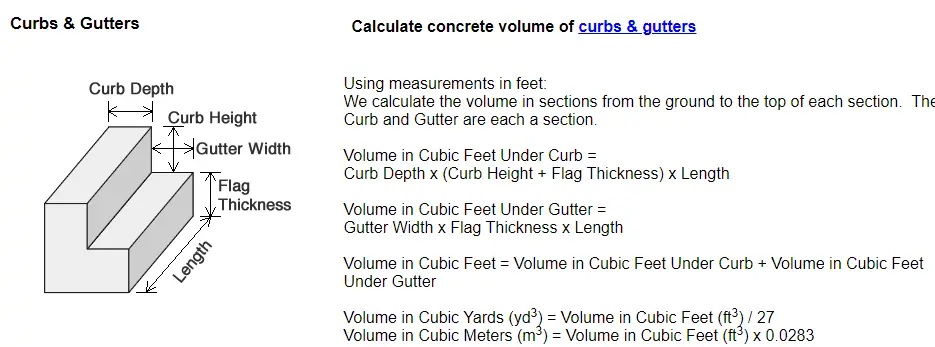When you use a formula to calculate concrete yardage for curb and gutter, it's very similar to steps.

You break it down into sections and calculate for each section, then add the sections together.

Example: Curb Depth 6", Curb Height 6", Gutter Width 18", Flag Thickness 1', Length 80'

(Convert all dimensions into feet) and calculate cubic feet for each section.

Curb = .5 x (.5 + 1) x 80 = 60 cubic feet

Gutter = 1.5 x 1 x 80 = 120 cubic feet

Total for cubic feet = 180 cubic feet

Cubic yards = cubic feet 180 ÷ 27 = 6.66 yd³ of concrete

Cubic Meters = cubic feet 180 x .0283 = 5.09 m³ of concrete

You can check yourself using this:

Concrete Calculator
One more way to share everything-about-concrete.com